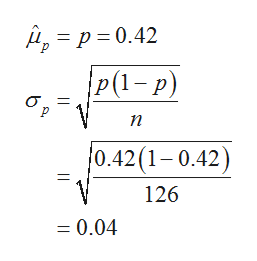A Food Marketing Institute found that 42% of households spend more than \$125 a week on groceries. Assume the population proportion is 0.42 and a simple random sample of 126 households is selected from the population. What is the probability that the sample proportion of households spending more than \$125 a week is between 0.2 and 0.39?Answer =

Question

A Food Marketing Institute found that 42% of households spend more than \$125 a week on groceries. Assume the population proportion is 0.42 and a simple random sample of 126 households is selected from the population. What is the probability that the sample proportion of households spending more than \$125 a week is between 0.2 and 0.39?

Step 1

It was found that 42% of the households spend more than \$125 on groceries.

That is, the population proportion, p=0.42.

A sample of 126 households is selected from the population. That is, the sample size n = 126.

Let p-hat denote the sample proportion of households spending more than \$125 on groceries.

In other words, the mean of the sampling distribution of p-hat is equal to the population proportion p and the standard deviation of the sampling distribution of p-hat is equal to sqrt[(p(1-p)/n].

Thus, the mean and standard deviation of the sample proportion of households spending more than \$125 on groceries is,help_outlineImage Transcriptionclosep 0.42 P(1-p) n 0.42(1-0.42) 11 126 = 0.04 fullscreen
Step 2

Computing the probability that the sample proportion of households spending more than \$125 a week on groceri...

Want to see the full answer?

See Solution

Want to see this answer and more?

Our solutions are written by experts, many with advanced degrees, and available 24/7

See Solution
Tagged in

Other